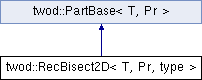# twod::RecBisect2D< T, Pr, type > Class Template Reference

implements variants of the recursive bisection algorithm originally published in "Marsha Berger and Shahid Bokhari, A Partitioning Strategy for Nonuniform Problems on Multiprocessors, IEEE TC, 1987". More...

`#include <twod/rec_bisect_2d.hpp>`

Inheritance diagram for twod::RecBisect2D< T, Pr, type >:## Detailed Description

### template<typename T, typename Pr, int type = 0> class twod::RecBisect2D< T, Pr, type >

implements variants of the recursive bisection algorithm originally published in "Marsha Berger and Shahid Bokhari, A Partitioning Strategy for Nonuniform Problems on Multiprocessors, IEEE TC, 1987".

Parameters:
 T is the data type of prefix sum array Pr is the 2D prefix sum array type is the recursive bisection strategy type. Strategy types are LOAD, DIST, VERT_ALT, HOR_ALT. Default is LOAD.

List of all members.

## Public Member Functions

virtual T part (int procCount, const Pr &prefixSumArray, util::RectList< T, Pr > &parts)
Applies a 2d partitioning algorithm to a given 2d prefixSumArray .
virtual ~RecBisect2D ()

## Static Public Member Functions

static T rec_bisect_2d_internal (int procCount, const Pr &prefixSumArray, util::RectList< T, Pr > &parts, util::rectangle r, bool orient)

## Static Public Attributes

static const int LOAD = 0
static const int DIST = 1
Cut to minimize side-to-side distance.
static const int VERT_ALT = 2
start vertically and alternate between horizontal
static const int HOR_ALT = 3
start horizontally and alternate between vertical

## Constructor & Destructor Documentation

template<typename T , typename Pr , int type = 0>
 virtual twod::RecBisect2D< T, Pr, type >::~RecBisect2D ( ) ` [inline, virtual]`

## Member Function Documentation

template<typename T , typename Pr , int type>
 T twod::RecBisect2D< T, Pr, type >::part ( int procCount, const Pr & prefixSumArray, util::RectList< T, Pr > & parts ) ` [virtual]`

Applies a 2d partitioning algorithm to a given 2d prefixSumArray .

Parameters:
 [in] procCount is the number of processors [in] prefixSumArray first column and first row consists of zero only. But the borders of rectangles in rect_list never touch this area (index 0 in row or column) [out] parts must be empty before calling this function. It will contain the partition after the function return
Returns:

Implements twod::PartBase< T, Pr >.

template<typename T , typename Pr , int type>
 T twod::RecBisect2D< T, Pr, type >::rec_bisect_2d_internal ( int procCount, const Pr & prefixSumArray, util::RectList< T, Pr > & parts, util::rectangle r, bool orient ) ` [static]`

## Member Data Documentation

template<typename T , typename Pr , int type = 0>
 const int twod::RecBisect2D< T, Pr, type >::DIST = 1` [static]`

Cut to minimize side-to-side distance.

template<typename T , typename Pr , int type = 0>
 const int twod::RecBisect2D< T, Pr, type >::HOR_ALT = 3` [static]`

start horizontally and alternate between vertical

template<typename T , typename Pr , int type = 0>
 const int twod::RecBisect2D< T, Pr, type >::LOAD = 0` [static]`

 const int twod::RecBisect2D< T, Pr, type >::VERT_ALT = 2` [static]`